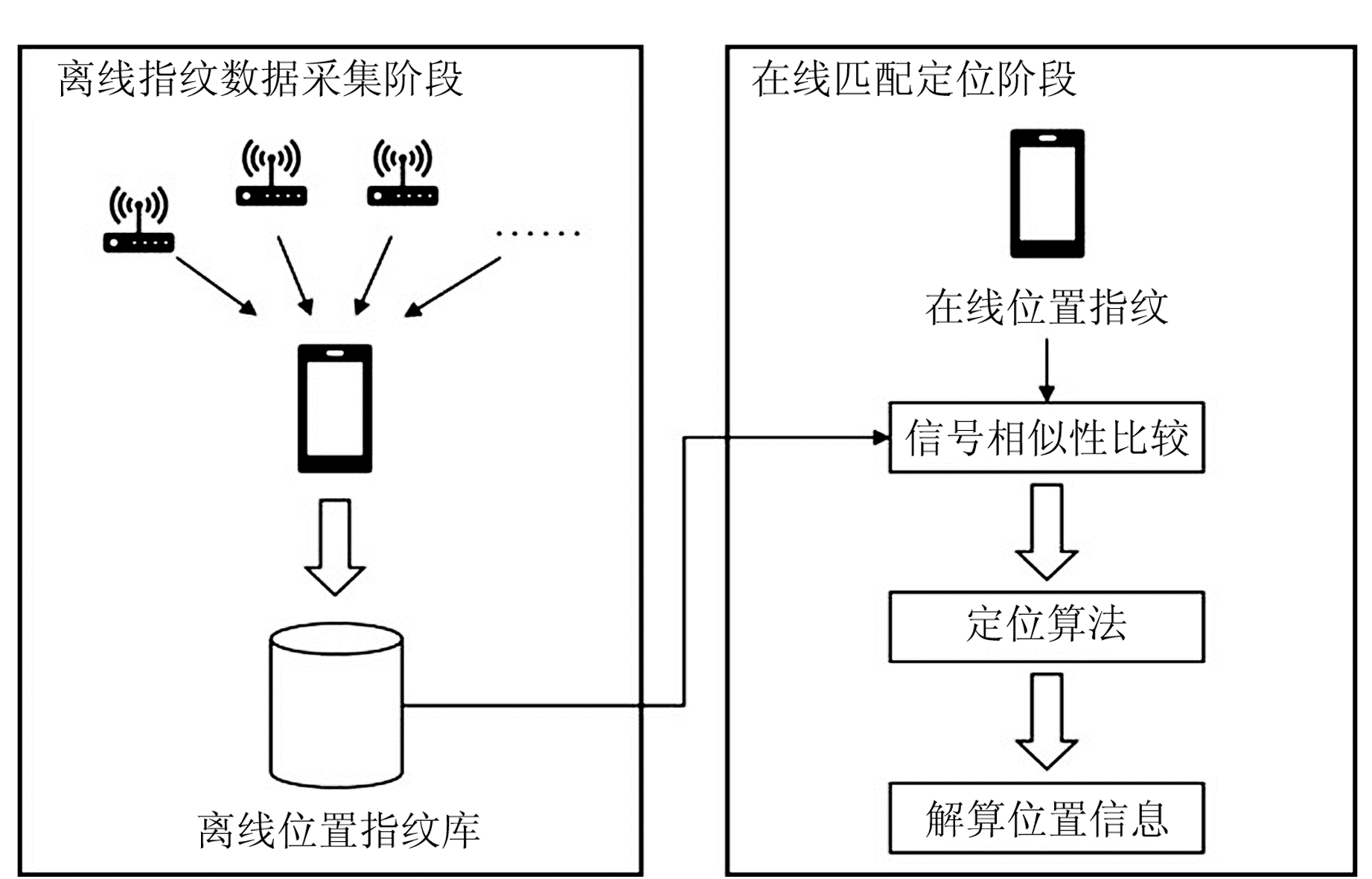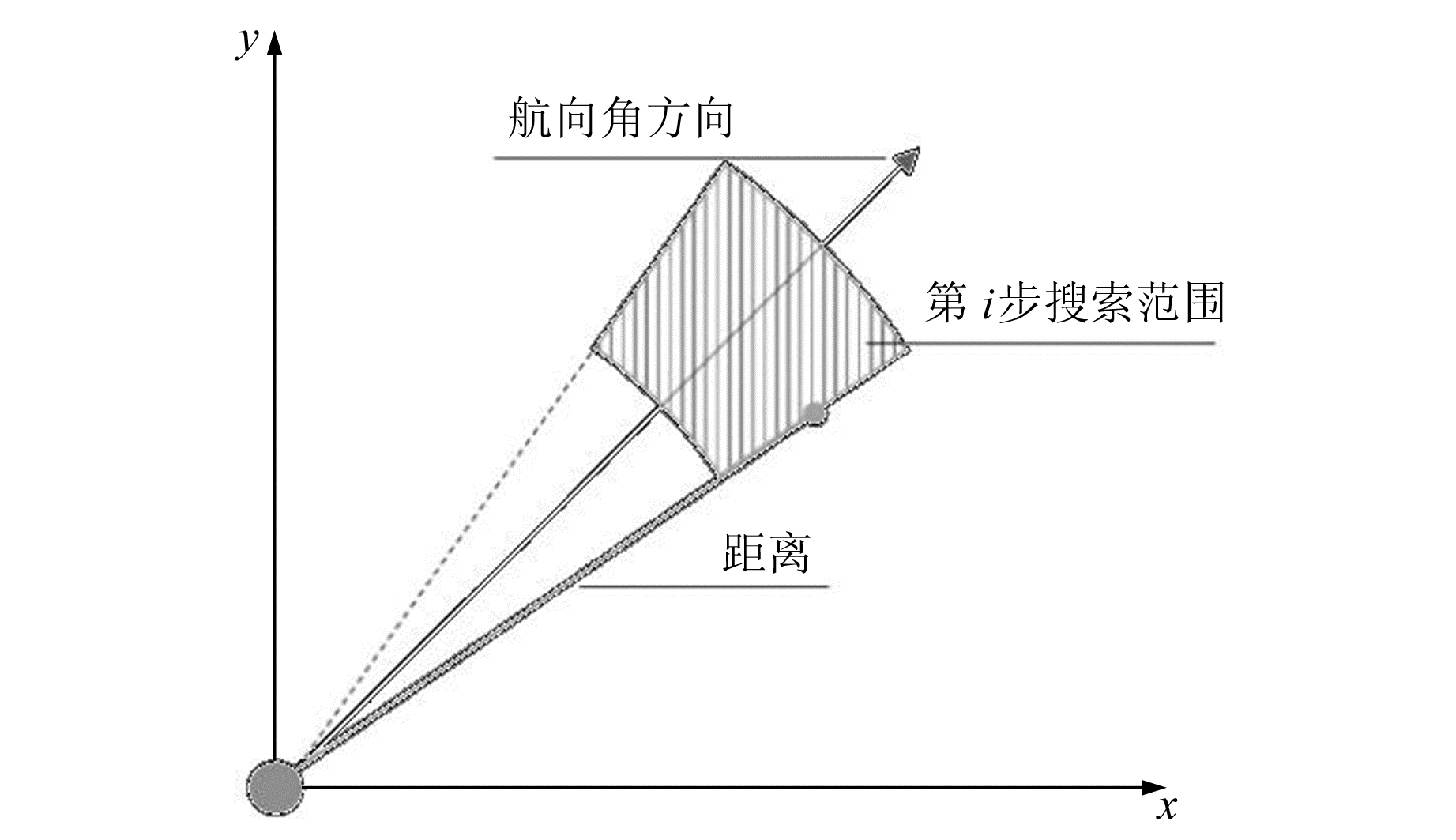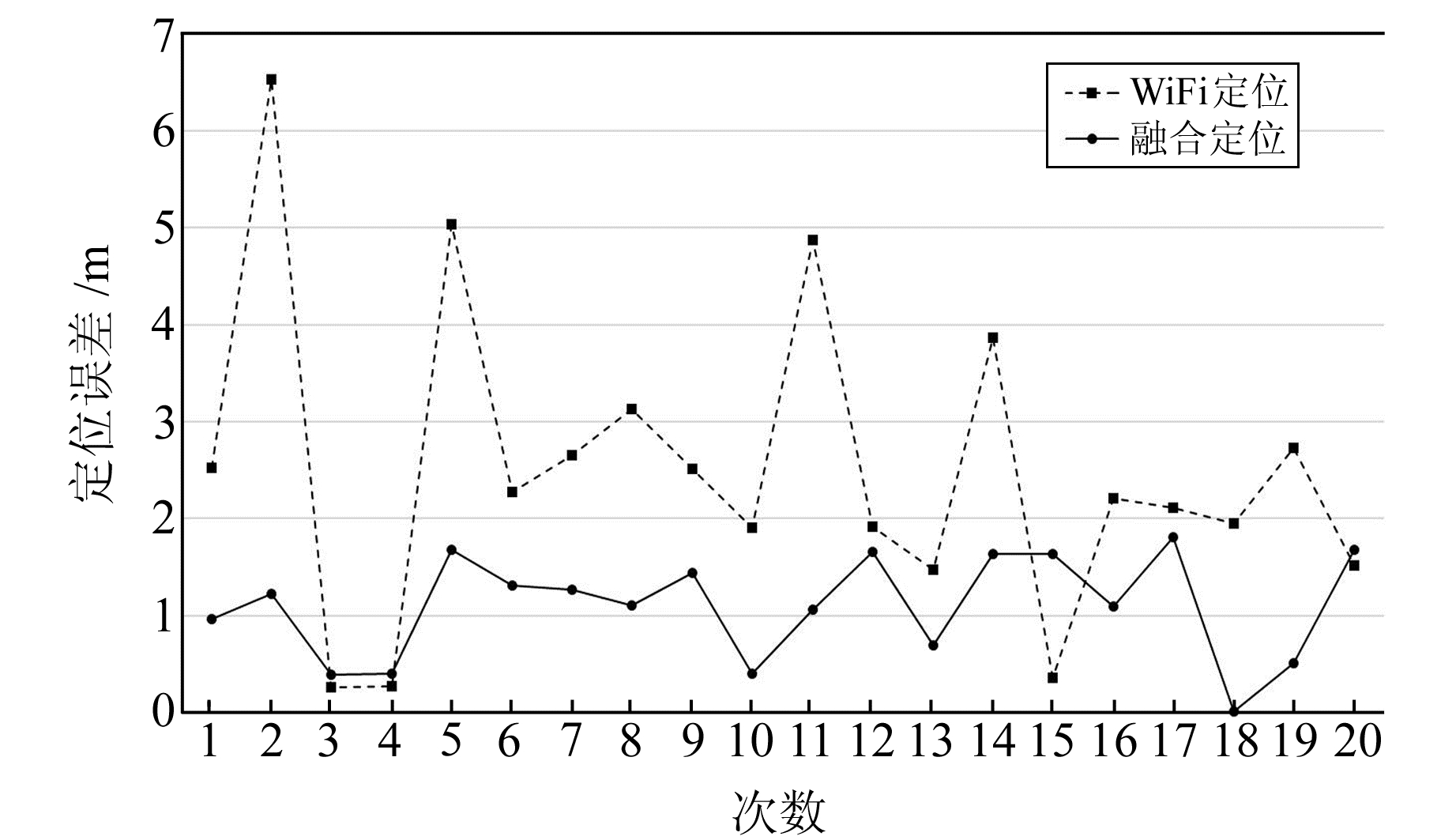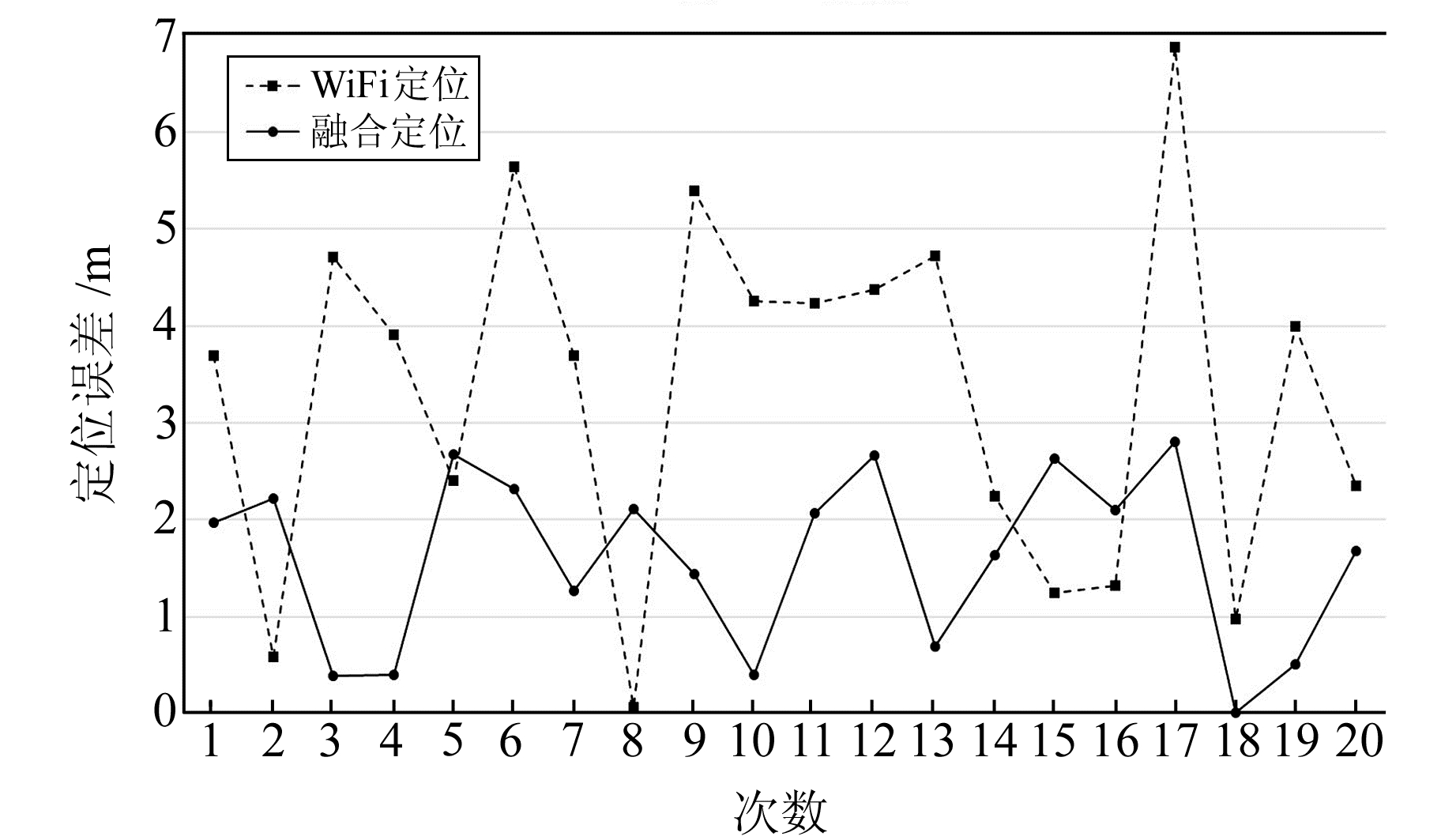﻿ 基于WiFi/PDR的室内定位初始位置融合解算方法研究文章快速检索 高级检索
 大地测量与地球动力学2019, Vol. 39Issue (6): 602-606  DOI: 10.14075/j.jgg.2019.06.010### 引用本文LI Mingfeng, WANG Shen, TAN Ding. Research on the Fusion Method of the Initial Position of Indoor Location Based on WiFi/PDR[J]. Journal of Geodesy and Geodynamics, 2019, 39(6): 602-606.### Foundation support

Key Research & Development Program of Jiangsu Province, No.BE2015698;Science and Technology Project of Nanjing, No.201716027.

### 第一作者简介

LI Mingfeng, PhD, professor, PhD supervisor, majors in deformation monitoring and disaster warning, 3S technology integration and application, E-mail: njuter@163.com.

### 文章历史

1. 南京工业大学测绘科学与技术学院，南京市浦珠南路30号，211816;
2. 武汉大学测绘学院，武汉市珞喻路129号，430079

1 基于WKNN的WiFi指纹定位法

WiFi指纹定位将待定点位置指纹与已知位置的所有离线位置指纹通过遍历比较2种指纹间的信号相似度，参照相似度最高的位置估算待定点位置。常用的待定点位置估计方法包括确定性算法、概率性算法和人工神经网络算法。其中确定性算法具有计算量小、存储量小等优势，适合在智能手机上使用。

1.1 WiFi指纹定位原理图 1 WiFi位置指纹定位原理 Fig. 1 Principle of WiFi location fingerprint positioning

n维向量AB的余弦相似度表示为:

 $\begin{array}{*{20}{c}} {{\mathop{\rm sim}\nolimits} (\mathit{\boldsymbol{A}}, \mathit{\boldsymbol{B}}) = \left[ {\sum\limits_{i = 1}^n {\left( {{A_i} \times {B_i}} \right)} } \right]/}\\ {\left[ {\sqrt {\sum\limits_{i = 1}^n {{{\left( {{A_i}} \right)}^2}} } \times \sqrt {\sum\limits_{i = 1}^n {{{\left( {{B_i}} \right)}^2}} } } \right]} \end{array}$ (1)

 ${\rm{f}}{{\rm{p}}_i} = \left\{ {\left( {{M_1}, {R_{i1}}} \right), \left( {{M_2}, {R_{i2}}} \right), \cdots , \left( {{M_n}, {R_{in}}} \right)} \right\}$ (2)

 ${{\rm{f}}{{{\rm{p}}_j}}} = \left\{ {\left( {{M_1}, {R_{j1}}} \right), \left( {{M_2}, {R_{j2}}} \right), \cdots , \left( {{M_n}, {R_{jn}}} \right)} \right\}$ (3)

 ${\rm{MA}}{{\rm{C}}_c} = \left\{ {{M_1}, {M_2}, \cdots , {M_k}} \right\}$ (4)

 $\begin{array}{*{20}{l}} {{\rm{f}}{{\rm{p}}_j}' = \left\{ {\left( {{M_1}, {R_{j1}}} \right), \left( {{M_2}, {R_{j2}}} \right), \cdots , \left( {{M_k}, {R_{jk}}} \right)} \right\}}\\ {{\rm{f}}{{\rm{p}}_i}' = \left\{ {\left( {{M_1}, {R_{i1}}} \right), \left( {{M_2}, {R_{i2}}} \right), \cdots , \left( {{M_k}, {R_{ik}}} \right)} \right\}} \end{array}$ (5)

k个离线指纹中第a个参考点权重值记为：

 ${w_a} = {{\mathop{\rm sim}\nolimits} _a}/\sum\limits_{b = 1}^k {{\rm{si}}{{\rm{m}}_b}}$ (6)

 $(\bar x, \bar y) = \sum\limits_{i = 1}^k {{w_a}} \left( {{x_a}, {y_a}} \right)$ (7)
2 行人航位推算定位法

PDR定位法是一种基于集成传感器完全自主式的惯性测量定位方法，本质上是一种利用相对位置关系定位的方法，其计算公式为：

 $\left\{ {\begin{array}{*{20}{l}} {{x_k} = {x_0} + \sum\limits_{i = 1}^k {{l_i}} \sin {\theta _i}}\\ {{y_k} = {y_0} + \sum\limits_{i = 1}^k {{l_i}} \cos {\theta _i}} \end{array}} \right.$ (8)

 ${a_c} = \sqrt {a_x^2 + a_y^2 + a_z^2}$ (9)

 ${\rm{PT}} = {\rm{PV}}/{\rm{PN}}$ (10)

 $l{\rm{ = }}a{\rm{ }}f + b$ (11)
 $l = K\sqrt{{{a_{\max }} - {a_{\min }}}}$ (12)

2种模型的平均误差分别为0.08 m和0.03 m，误差均在cm级，对定位精度的影响基本可忽略。但线性频率模型计算量远小于Weinberg Approach模型，因此，选择前者作为步长估算模型。

 $\left\{ {\begin{array}{*{20}{l}} {{\theta _{{Z_X}}} = \frac{{{g_x}\theta }}{{\sqrt {g_x^2 + g_y^2 + g_z^2} }} = \frac{{{g_x}{\theta _x}}}{g}}\\ {{\theta _Z} = {\theta _{{Z_X}}} + {\theta _{{Z_Y}}} + {\theta _{{Z_Z}}}} \end{array}} \right.$ (13)

 $A = {A_0} + \sum\limits_{i = 1}^k {{\theta _{{Z_i}}}}$ (14)
3 基于WiFi/PDR的初始位置融合解算方法 3.1 初始位置解算方法

 $n = 1 + ft$ (15)

 ${S_i} = \left\{ {\left( {{x_{i1}}, {y_{i1}}} \right), \left( {{x_{i2}}, {y_{i2}}} \right), \cdots , \left( {{x_{in}}, {y_{in}}} \right)} \right\}$ (16)

Si-1n个点与Sin个候选点分别进行距离和方位计算，以评估已有轨迹行走到当前WiFi定位点的可能性。将Si-1中第m个点到Si中第j个参考点之间的距离记为lmj，连接线形成的向量与磁北极的夹角记为Amj，顺时针方向记为正方向，范围为(-180°，180°]。lmjAmj具体可由式(17)求得：

 $\begin{array}{l} \\ \left\{ \begin{array}{l} {l_{mj}} = \sqrt {{{\left( {{x_{ij}} - {x_{(i - 1)m}}} \right)}^2} + {{\left( {{y_{ij}} - {y_{(i - 1)m}}} \right)}^2}} \\ {A_{mj}}{\rm{ = }}\left\{ \begin{array}{l} \arctan \left( {\frac{{{y_{ij}} - {y_{(i - 1)m}}}}{{{x_{ij}} - {x_{(i - 1)m}}}}} \right), {x_{ij}} - {x_{(i - 1)m}} \ne 0\\ 0, {x_{ij}} - {x_{(i - 1)m}} = 0, {y_{ij}} - {y_{(i - 1)m}} > 0\\ \pi , {x_{ij}} - {x_{(i - 1)m}} = 0, {y_{ij}} - {y_{(i - 1)m}} < 0 \end{array} \right. \end{array} \right. \end{array}$ (17)

 $\Delta l = {l_{mj}} - l$ (18)

 $\Delta A = {A_{mj}} - A$ (19)

 $\left\{ \begin{array}{l} {p_l} = \frac{{{{\rm{e}}^{ - \frac{{\Delta l}}{{2\sigma _l^2}}}}}}{{\sqrt {2\pi } {\sigma _l}}}\\ {p_A} = \frac{{{{\rm{e}}^{ - \frac{{\Delta A}}{{2\sigma _A^2}}}}}}{{\sqrt {2\pi } {\sigma _A}}} \end{array} \right.$ (20)图 2 定位坐标限定范围 Fig. 2 Limited scope of positioning coordinates

 ${p_U} = {p_l}{p_A}$ (21)

 $\Delta A = {A_{i + 1}} - {A_i}$ (22)

3.2 实验及分析图 3 理想定位场景下WiFi定位方法与融合解算方法估算误差 Fig. 3 Estimation errors of WiFi location and fusion solution in ideal location scene表 1 理想定位场景下不同定位方法误差分布 Tab. 1 Error distribution of different locating methods in ideal location scene表 2 复杂定位场景下不同定位方法误差分布 Tab. 2 Error distribution of different locating methods in complex location scene图 4 复杂定位场景下WiFi定位方法与融合解算方法估算误差 Fig. 4 Estimation errors of WiFi location and fusion algorithm in complex location scene
4 结语

1) 针对WiFi指纹定位中设备差异性问题，采用余弦相似度比较信号相似度，并以此为权值实现WKNN定位算法。

2) 针对任意姿态下智能手机的PDR步数检测和航向角估计问题，分别提出基于综合线性加速度的平均阈值步数检测法和基于重力加速度的坐标转换法，以3 s为周期计算时间段内波峰对应综合线性加速度值的均值，并以此为阈值过滤无效波峰，结合相邻两步间的最小时间间隔，实现精准步数检测；基于陀螺仪数据，以重力加速度为纽带，实现手机任意姿态下手机坐标系到行人坐标系的转换，为航向角计算奠定基础。

3) 针对融合定位中初始位置不精准的问题，在研究WiFi定位、PDR定位实现方法的基础上，提出基于WiFi/PDR的初始位置融合解算方法。该方法定位精度为1.2~1.8 m，相比WiFi指纹定位误差缩小50%左右，且波动稳定，误差均小于3 m，为提供更准确、有效的室内定位服务奠定了基础。

  姚碧超.室内WiFi定位技术研究[D].成都: 电子科技大学, 2017 (Yao Bichao. Research on Indoor Location Technology Based on WiFi[D]. Chengdu: University of Electronic Science and Technology, 2017) http://cdmd.cnki.com.cn/Article/CDMD-10614-1017077591.htm (0)  周飞.室内WiFi定位的研究[D].成都: 西南财经大学, 2016 (Zhou Fei. Study on Indoor WiFi Localization[D]. Chengdu: Southwest University of Finance and Economics, 2016)) http://cdmd.cnki.com.cn/Article/CDMD-10651-1017016717.htm (0)  黄承恺.多传感器信息融合算法在室内定位中的应用[D].北京: 北京邮电大学, 2015 (Huang Chengkai. The Application of Multi-Sensor Information Fusion Algorithm for Indoor Positioning System[D]. Beijing: Beijing University of Posts and Telecommunications, 2015) (0)  李冬, 张宝贤. 基于指纹的室内定位技术[J]. 中兴通讯技术, 2015, 21(6): 31-34 (Li Dong, Zhang Baoxian. Techniques for Fingerprint Based Indoor Localization[J]. ZTE Technology Journal, 2015, 21(6): 31-34 DOI:10.3969/j.issn.1009-6868.2015.06.008) (0)  殷虎. WLAN位置指纹室内定位技术[D].长沙: 湖南大学, 2016 (Yin Hu. An Indoor Localization Method Based on Location Fingerprinting in WLAN[D]. Changsha: Hunan University, 2016) http://cdmd.cnki.com.cn/Article/CDMD-10532-1016252375.htm (0)  王珊.基于惯性传感器和WiFi联合室内定位方法的研究与实现[D].南京: 南京邮电大学, 2015 (Wang Shan. The Study and Implementation of Indoor Positioning Based on Inertial Sensors and WiFi[D]. Nanjing: Nanjing University of Posts and Telecommunications, 2015) http://d.wanfangdata.com.cn/Periodical/zxtxjs201506008 (0)  Mladenov M, Mock M. A Step Counter Service for Java-Enabled Devices Using a Built-in Accelerometer[C]. The 1st International Workshop on Context-Aware Middleware and Services: Affiliated with the 4th International Conference on Communication System Software and Middleware, Dublin, 2009 http://dl.acm.org/citation.cfm?id=1554235 (0)  Li F, Zhao C S, Ding G Z, et al. A Reliable and Accurate Indoor Localization Method Using Phone Inertial Sensors[C]. 2012 ACM Conference on Ubiquitous Computing, Pittsburgh, 2012 https://www.researchgate.net/publication/262211952_A_Reliable_and_accurate_indoor_localization_method_using_phone_inertial_sensors (0)  Weinberg H. Using the ADXL202 in Pedometer and Personal Navigation Applications[J]. Analog Devices AN-602 Application Note, 2002, 2(2): 1-6 (0)  Lee S, Chon Y, Cha H. Smartphone-Based Indoor Pedestrian Tracking Using Geo-Magnetic Observations[J]. Mobile Information Systems, 2013, 9(2): 123-137 DOI:10.1155/2013/295838 (0)
Research on the Fusion Method of the Initial Position of Indoor Location Based on WiFi/PDR
LI Mingfeng1WANG Shen1     TAN Ding2
1. Institute of Surveying Science and Technology, Nanjing Tech University, 30 South-Puzhu Road, Nanjing 211816, China;
2. School of Geodesy and Geomatics, Wuhan University, 129 Luoyu Road, Wuhan 430079, China
Abstract: This paper proposes a fusion method of initial position based on WiFi/PDR. In this method, the WiFi positioning results within 0.2 s after the steps are detected are set, the adjacent two-step distances and heading angles estimated in the PDR are taken as reference, the adjacent two-step WiFi positioning results are screened by the distance and angle thresholds, and the optimal positioning results are chosen according to the degree of deviation from the angle. Experimental results show that the initial position accuracy of this method is 1.2-1.8 m, which is 50% smaller than WiFi fingerprint positioning error.
Key words: indoor location; WiFi fingerprint location; pedestrian dead reckoning; fusion location